# Homology functor

Jump to: navigation, search

A functor on an Abelian category that defines a certain homological structure on it. A system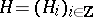of covariant additive functors from an Abelian categoryinto an Abelian categoryis called a homology functor if the following axioms are satisfied.

1) For each exact sequence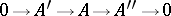and each, ina morphism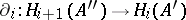is given, which is known as the connecting or boundary morphism.

2) The sequence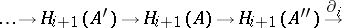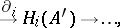called the homology sequence, is exact.

Thus, let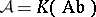be the category of chain complexes of Abelian groups, and letbe the category of Abelian groups. The functorswhich assign to a complexthe corresponding homology groups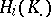define a homology functor.

Let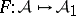be an additive covariant functor for which the left derived functors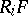(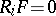,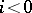) are defined (cf. Derived functor). The systemwill then define a homology functor frominto.

Another example of a homology functor is the hyperhomology functor.

A cohomology functor is defined in a dual manner.